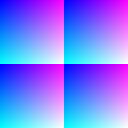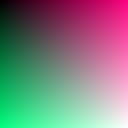Each colour on your computer screen is composed by a certain amount of red, green and blue. These amounts vary from zero (no colour, black) upto 255 (full colour).

For example, mixing red and green makes some yellow, and yellow's definition is (255,255,0): full red and green and no blue.
You have probably already seen those red,green and blue sliders in paint software.
Here is a small list of colours and their definitions:

(0,0,0) is black and (255,255,255) is white.
(255,0,0) is red, (0,255,0) is green and (0,0,255) is blue.
(0,255,255) is cyan, (255,0,255) is magenta and (255,255,0) is yellow.

Now open a new project window if none is opened yet ( 'NEW' button)

The three fields named r(0,x,y)=, g(0,x,y)= and b(0,x,y)= [zero may be another number, depending on the current project].
contains these definitions. So if you write:
r(0,x,y)=255 (copy only what is in bold)
g(0,x,y)=255
b(0,x,y)=0

And click on "render", the current project window will fill with yellow. You may try to put other numbers.

Instead of just numbers, you may put functions of x and y. X is the number of pixels from the left of the window, beginning from zero, and y is the number of pixels from the top.
Try now setting:

 r(0,x,y)=x g(0,x,y)=y b(0,x,y)=255You will see a nice spread. In the top left of the window, x=y=0 and the colour components is (0,0,255), blue.
In the top right corner, x is 255, and the components will be (255,0,255), which is magenta.
In the bottom border, it goes from (0,255,255) (cyan) to (255,255,255) (white). Try clicking in some places of the project window and look what is written in the bottom part of the messages-window.

· The computer automatically applies a 256 modulo to avoid overflowing. Try the following to see it:

 r(0,x,y)=2*x g(0,x,y)=2*y b(0,x,y)=255Let's try some more complex operations:

The following are the default settings.

 r(0,x,y)=x g(0,x,y)=y b(0,x,y)=(x+y)/2Try clicking in some places of the project window after having rendered, and look in the message window..

In the second chapter we will do more theory than pratic...

Index

Chapter one: Introduction

1.2: What can't be done with picFX